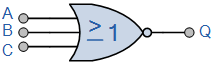Thursday, March 02, 2017

Logic NOR Gate
The Logic NOR Gate or Inclusive-NOR gate is a combination of the digital logic OR gate with that of an inverter or NOT gate connected together in series.

The NOR (Not – OR) gate has an output that is normally at logic level “1” and only goes “LOW” to logic level “0” when ANY of its inputs are at logic level “1”. The Logic NOR Gate is the reverse or “Complementary” form of the OR gate we have seen previously.

Logic NOR Gate EquivalentThe logic or Boolean expression given for a logic NOR gate is that for Logical Multiplication which it performs on the complements of the inputs. The Boolean expression for a logic NOR gate is denoted by a plus sign, ( + ) with a line or Overline, ( ‾‾ ) over the expression to signify the NOT or logical negation of the NOR gate giving us the Boolean expression of:  A+B = Q.
Then we can define the operation of a 2-input digital logic NOR gate as being:
“If both A and B are NOT true, then Q is true”

Transistor NOR Gate

A simple 2-input logic NOR gate can be constructed using RTL Resistor-transistor switches connected together as shown below with the inputs connected directly to the transistor bases. Both transistors must be cut-off “OFF” for an output at Q.Logic NOR Gates are available using digital circuits to produce the desired logical function and is given a symbol whose shape is that of a standard OR gate with a circle, sometimes called an “inversion bubble” at its output to represent the NOT gate symbol with the logical operation of the NOR gate given as.

The Digital Logic “NOR” Gate

Symbol             Truth Table3-input NOR Gate C B A Q 0 0 0 1 0 0 1 0 0 1 0 0 0 1 1 0 1 0 0 0 1 0 1 0 1 1 0 0 1 1 1 0 Boolean Expression Q = A+B+C Read as A OR B OR C gives NOT Q

As with the OR function, the NOR function can also have any number of individual inputs and commercial available NOR Gate IC’s are available in standard 2, 3, or 4 input types. If additional inputs are required, then the standard NOR gates can be cascaded together to provide more inputs for example.

A 4-input NOR FunctionThe Boolean Expression for this 4-input NOR gate will therefore be:   Q = A+B+C+D
If the number of inputs required is an odd number of inputs any “unused” inputs can be held LOW by connecting them directly to ground using suitable “Pull-down” resistors.
The Logic NOR Gate function is sometimes known as the Pierce Function and is denoted by a downwards arrow operator as shown, AB.

The “Universal” NOR Gate

Like the NAND gate seen in the last section, the NOR gate can also be classed as a “Universal” type gate. NOR gates can be used to produce any other type of logic gate function just like the NAND gate and by connecting them together in various combinations the three basic gate types of AND, OR and NOT function can be formed using only NOR‘s, for example.

Various Logic Gates using only NOR GatesAs well as the three common types above, Ex-Or, Ex-Nor and standard NOR gates can also be formed using just individual NOR gates.
Commonly available digital logic NOR gate IC’s include:
TTL Logic NOR Gates